# TOPIC: UNDERSTANDING 2D CONSTRIANTS IN AUTODESK INVENTOR® - PART 1

## INTRODUCTION

Some of the questions that are usually asked by new Inventor users who are migrating from AutoCAD are:

1. How to do I perform object snap in Inventor?
2. How to do I perform object snap tracking?
3. How to do I calculate rectangular and polar coordinates in Inventor? Etc.

Well, in the lesson, I'll try to answer these questions.

The first thing you have know about Inventor is that it is a 3D parametric design software. It is called a parametric modelling software because the sizes of the geometry and the relationship of between the geometry in your sketches, the height or extent of your extrude feature, the relationship between parts in an assembly, etc, are all controlled and driven by parameters.

In AutoCAD, the familiar and common workflow is to create precise geometry by using the commands on the Draw panel or toolbar. So if you want a horizontal line of say 200mm, you have to use polar tracking to set the direction of the line to either 0 or 180 degrees, and then type the precise length of 200. So you must always create precise geometry from the word "go." When dimensions (AutoCAD) are placed in the drawing, the dimensions merely report the size of the line. You cannot use the dimension to control the length of the line. In AutoCAD 3D, you use 3D modifying tools like 3D Move, 3D Rotate, and 3D Align to assembly various parts to form an assembly. AutoCAD does not associate or define any strict relationship between those parts as they are being assembled.

However, in Autodesk Inventor, the story changes. In Inventor sketch environment, you do not need to bother yourself by creating precise geometry from the start. That is why it is called "a sketch." You simply sketch approximate sizes and orientations of your geometry and then use Inventor 2D Constraints to define strict sizes and relationship between the sketch geometry. In the assembly environment, you use Inventor 3D constraints to define strict relationships between parts, or components in the assembly. This way, the sketch, model, or assembly is stable, and cannot be easily rearrange or scattered deliberately or inadvertently. The only to rearrange or edit the sketch, model, or assembly is to edit or redefine the constraints that were applied to the sketch geometry or part (or components). These constraints that help define these sizes and relationships are known as parameters.

It is worth noting that parametric design was introduced into AutoCAD from AutoCAD 2010. So, you can redefine constraints in AutoCAD just like you can do in Inventor. But I can tell you, you may use them easily unless you have worked with a parametric design application like Inventor.

In Autodesk Inventor, we have two types of constraints, namely:

1. 2D constraints, and
2. 3D constraints.

2D constraints are only available in the Sketch Environment. They are used to define the sizes of geometry and the relationship between them in the Sketch Environment - which is Autodesk Inventor 2D environment.

3D constraints are only available in the Assembly Environment. They are used to define the relationship between parts, or components in an assembly.

## OBJECTIVES

At the end of this lesson, the reader should be able to:

1. Explain the concept of constraints in Autodesk Inventor
2. Differentiate between drawing in AutoCAD and sketching in Inventor.
3. Explain the types of 2D constraints.

## USING 2D CONSTRAINTS IN THE SKETCH ENVIRONMENT

Before we continue, Let me say, for the records, that 2D constraints can perform what Object Snaps, Object Snap Tracking, and Polar Tracking does in AutoCAD and even more. By the time you begin sketching with 2D constraints in Autodesk Inventor, you will be amazed by their sheer power! So let's continue.

There are two types of 2D constraints in Autodesk Inventor, namely:

1. Dimensional constraints, and
2. Geometric constraints.

Dimensional constraints are simply parametric dimension. The dimensions drive or determine the sizes and orientations of geometry in the sketch. For example dimensional constraints (or simply dimensions) can set a line to be 200mm. Nothing can make that line become 200.0001 mm unless the parametric dimension is edited. You can also apply a dimension that makes the angle between two lines to be 60 degrees.

Geometric constraints defines the geometric relationship between geometry in the sketch. For example, an horizontal line, a circle centered at the endpoint of a line geometry, a line that is perpendicular to another line, or a circle that is tangent to another circle.

The goodies for working with 2D constraints in the Inventor Sketch Environment are located on the Sketch tab > Constrain panel. In this lesson, we are going to focus on Geometric Constraints only. Dimensional constraints will be treated in the second part of this lesson.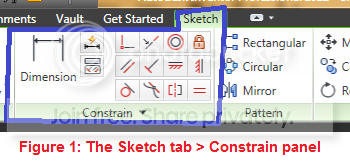We have the following geometric constraints in Inventor: Coincident constraint, Collinear constraint, Concentric constraint, Fixed Constraint, Parallel constraint, Perpendicular constraint, Horizontal constraint, Vertical constraint, Tangent constraint, Smooth constraint, Symmetric constraint, and Equal constraint.

The dimensional constraint is simply the Dimension tool.

### OVERVIEW OF THE GEOMETRIC CONSTRAINTS

1. COINCIDENT CONSTRAINT.

Symbol: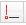Access: Sketch tab > Constrain panel >The coincident constraint is use to constrain a point on a geometry to touch other geometry in your sketch. For example, you may want to constraint the endpoint of an horizontal line to meet the center of a circle. The coincident constraint glyph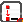is shown at the point where the endpoint of the line coincides with the center of the circle.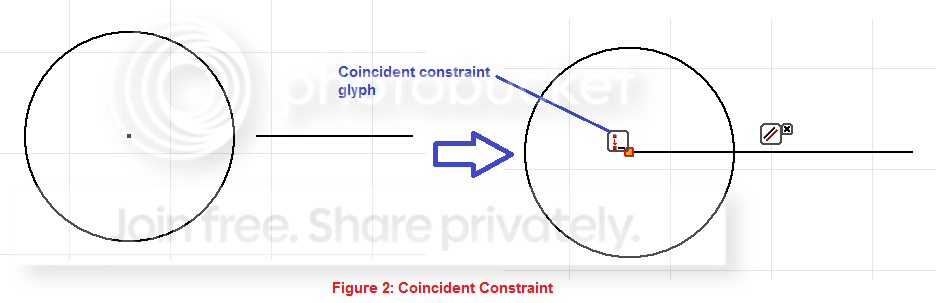HOT TIP: To see the constraints that are currently applied to the geometry in your sketch, simply right click in your sketch and click Show All Constraints on the short-cut menu, or press F8 on your keyboard. The constraints are displayed as glyphs. When you hover your cursor over a glyph, the sketch geometry associated with that glyph are highlighted.

2. COLLINEAR CONSTRAINT.

Symbol: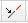Access: Sketch tab > Constrain panel >The collinear constraint constrains two or more line segments or ellipse axis to lie on the same line. In Figure 3, the top-most line is constrained to be collinear with the right-hand line of the closed geometry.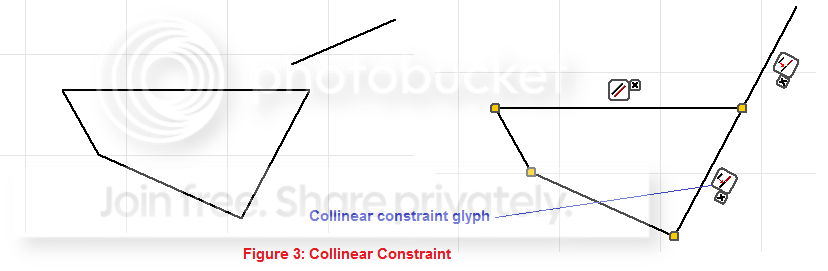3. CONCENTRIC CONSTRAINT.

Symbol: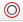Access: Sketch tab > Constrain panel >The concentric constraint is used to define a concentric relationship between any two circles or arcs. Concentricity means that both circles or arcs have the same centerpoint. In Figure 4, the 2-diameter circle is constrained to be concentric with the 4-diameter circle.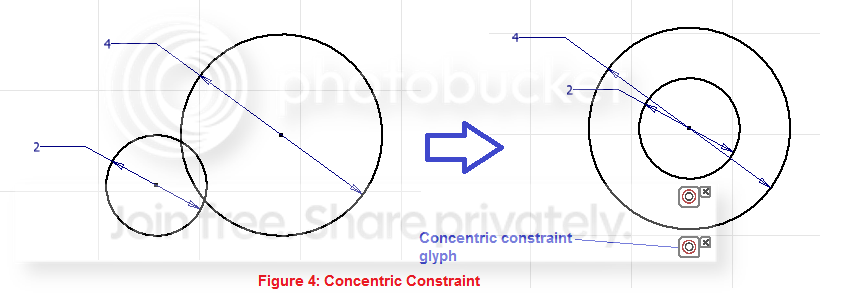4. FIXED CONSTRAINT.

Symbol: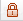Access: Sketch tab > Constrain panel >The fixed constraint is used to fixes points and curves in position relative to the sketch coordinate system. For example, when you apply fixed constraint to a circle, the circle can no longer be translated. That is, its position is fixed relative to the sketch coordinate system.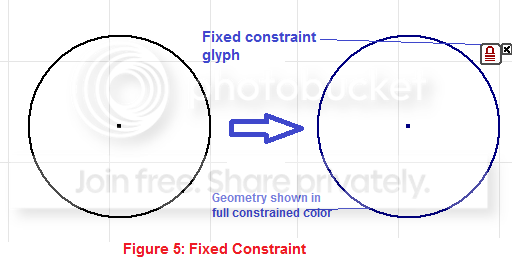5. PARALLEL CONSTRAINT.

Symbol: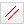Access: Sketch tab > Constrain panel >The parallel constraint is used to define parallel relationships between line segments in your sketch. In Figure 6, parallel constraints are applied to opposite lines in the sketch in addition to a horizontal constraint applied to the top line.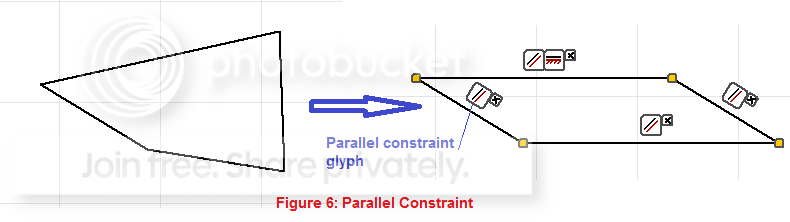6. PERPENDICULAR CONSTRAINT.

Symbol: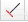Access: Sketch tab > Constrain panel >The perpendicular constraint is used to define perpendicularity between two lines. In Figure 7, the inclined line is constrained to be perpendicular to the horizontal line.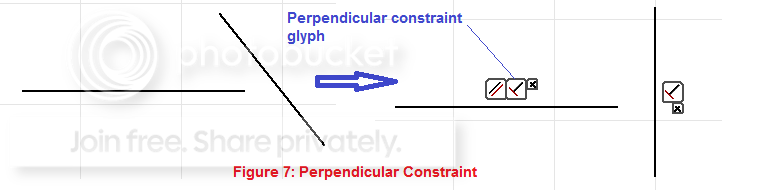7. HORIZONTAL CONSTRAINT.

Symbol: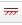Access: Sketch tab > Constrain panel >The horizontal constraint is used to constrain lines, ellipse axes, and pairs of points to lie parallel to the X axis of the sketch coordinate system. In Figure 8, horizontal constraint is applied to the left line. Horizontal constraint is also applied to constrain the left endpoint of the right line horizontally to the midpoint of the right-hand line of the rectangle.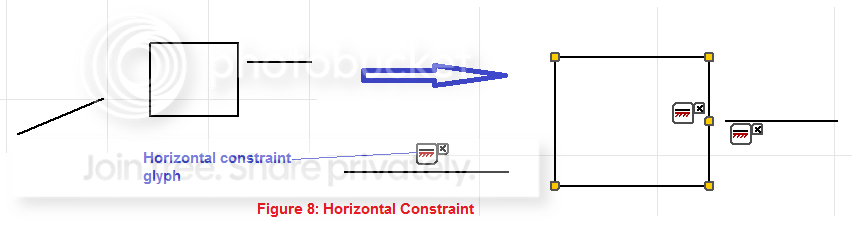8. VERTICAL CONSTRAINT.

Symbol: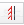Access: Sketch tab > Constrain panel >The vertical constraint is used to constrain lines, ellipse axes, and pairs of points to lie parallel to the Y axis of the sketch coordinate system. In Figure 9, a vertical constraint is applied to constrain the top vertex of the triangle to be vertical with the midpoint of the lower line of the triangle.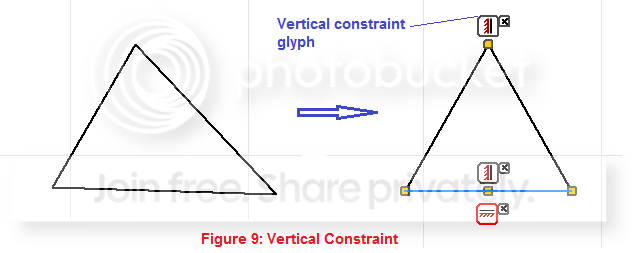9. TANGENT CONSTRAINT.

Symbol: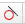Access: Sketch tab > Constrain panel >The tangent constraint is used to constrain lines, arcs, ellipses, ends of splines, and other geometry to be tangent to other geometry in the sketch. Figure 10 demonstrates the capability of the tangent constraint with the help of other constraints. It is quite interesting to see how the four disjointed geometry have been brought together parametrically through constraints.

This is a how the geometry to the right of Figure 10 was achieved.
1. Coincident constraint applied between arc 1 and circle, and also between arc 2 and circle.
2. Tangent constraint applied between arc 1 and circle, and also between arc 2 and circle.
3. Horizontal constraint applied between centerpoint of arc 1 and centerpoint of circle, and also applied between centerpoint of arc 2 and centerpoint of circle.
4. Equal constraint applied between arc 1 and arc 2.
5. Coincident constraint applied between the free endpoint of arc 1 and the left endpoint of the line.
6. Tangent constraint applied between arc 1 and the line.
7. Step v and vi repeated for arc 2 and the line.
8. The line is dragged upwards above the circle.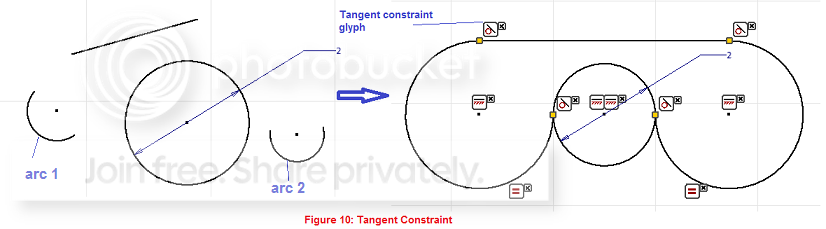10. SMOOTH CONSTRAINT.

Symbol: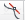Access: Sketch tab > Constrain panel >The smooth constraint is used to apply a G2 continuity condition between a spline and a line or arc. G2 continuity condition smoothens out the curvature of the spline as it joins the line. Smooth constraint is important during complex-shape design which can be done with Inventor or better with Autodesk Alias Design.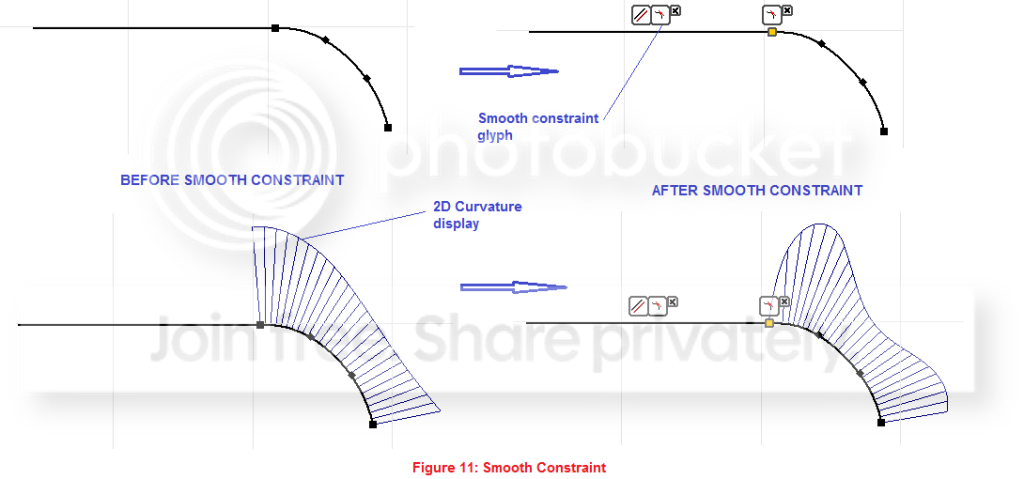11. SYMMETRIC CONSTRAINT.

Symbol: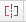Access: Sketch tab > Constrain panel >The symmetric constraint is used to constrain curves to be symmetrical to other curves about a line of symmetry - which could be a line geometry or a work axis. Symmetric constraint is also established between curves when you use the Mirror tool. In Figure 12, symmetric constraint is applied between the two circle with the center horizontal line as the line of symmetric, also between the two arcs with the center vertical line as the axis of symmetry.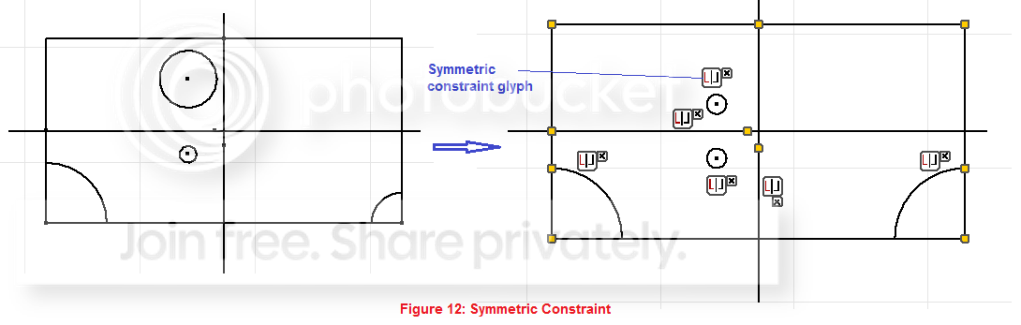12. EQUAL CONSTRAINT.

Symbol: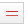Access: Sketch tab > Constrain panel >The equal constraint is used to constrain one geometry to be equal in size to another geometry in your sketch. Equal constraint is inferred when you use the symmetric constraint.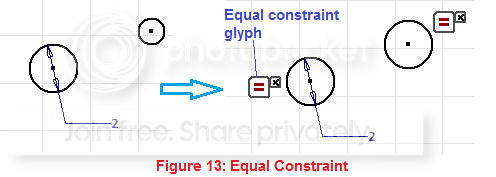In the next lesson - Understanding Constraints in Autodesk Inventor - Part 2, we are going to do a little practical on what we have learnt in this lesson.